Ex 7.3

Chapter 7 Class 6 Fractions
Serial order wise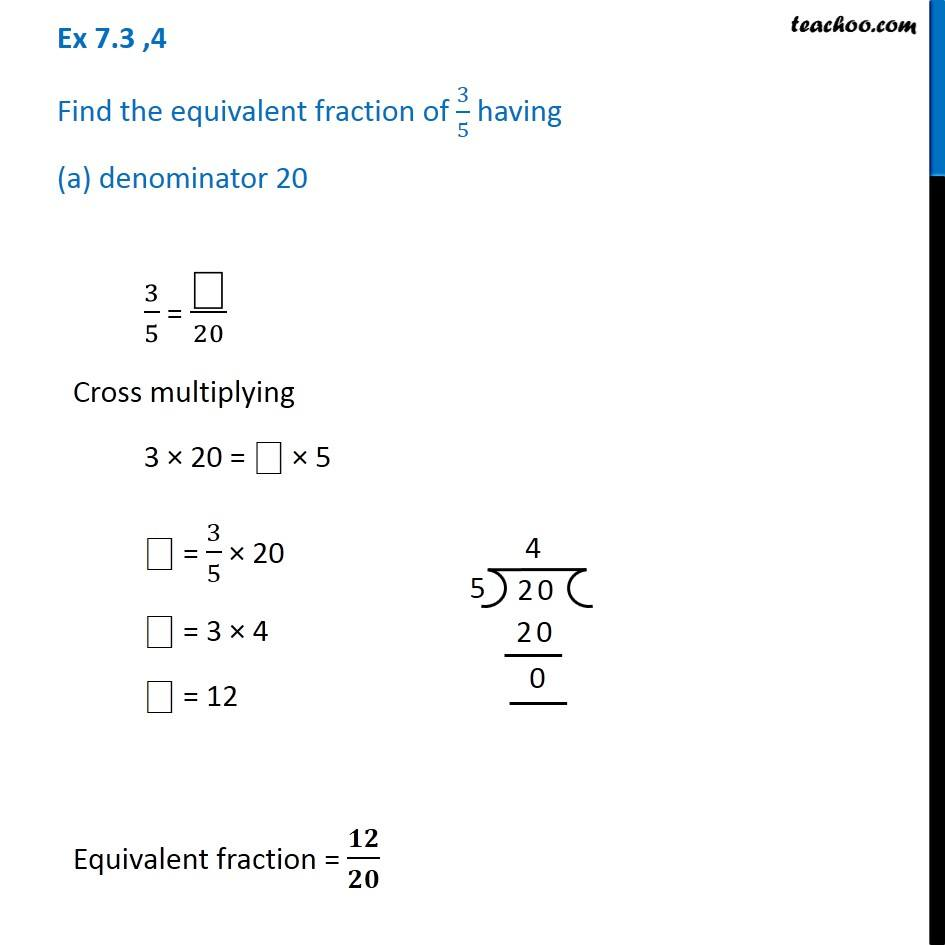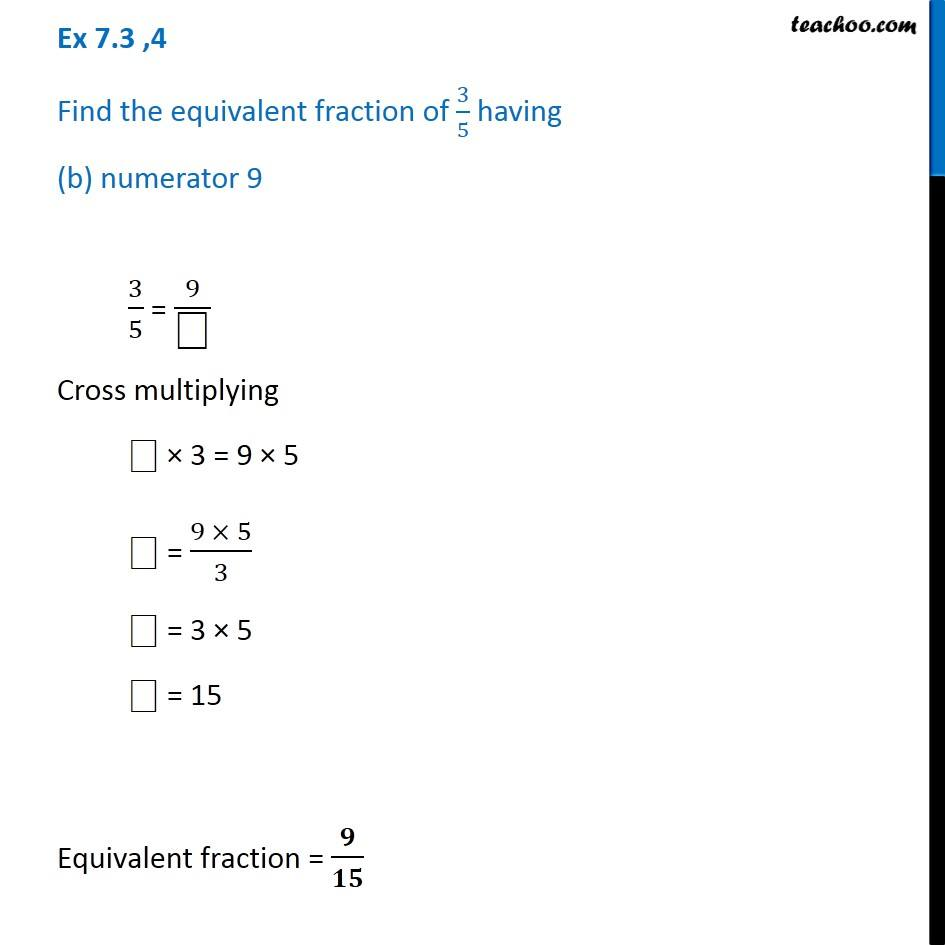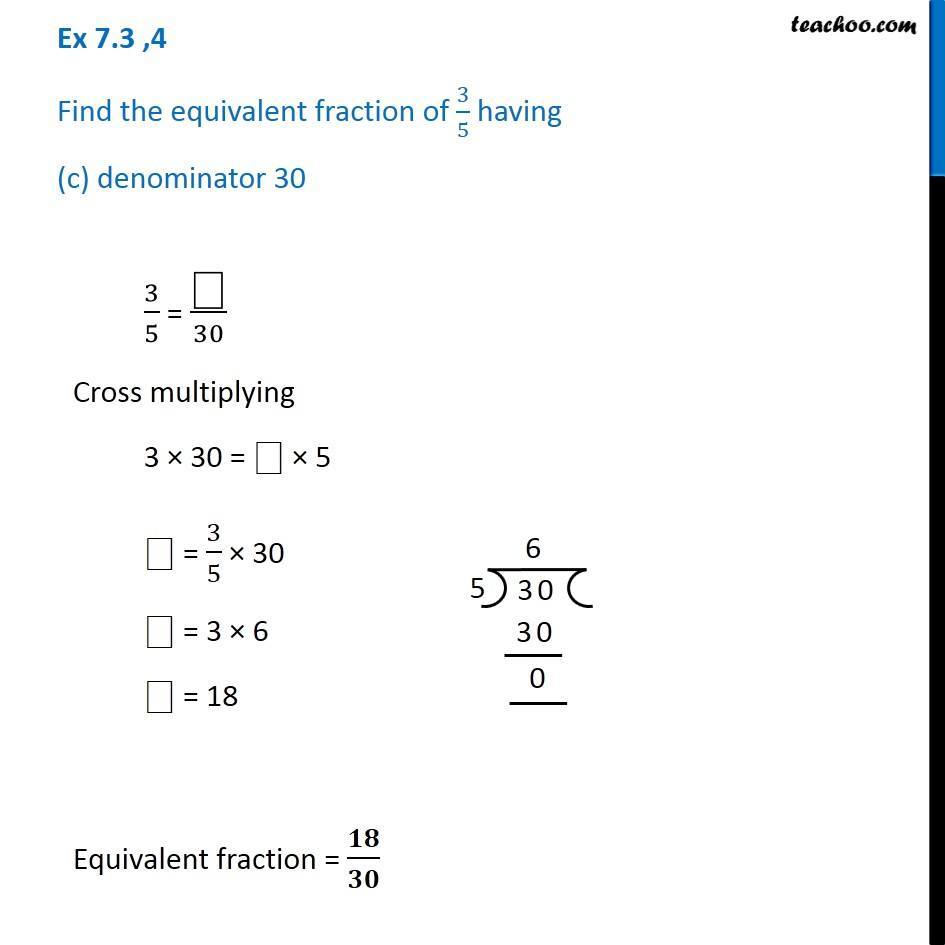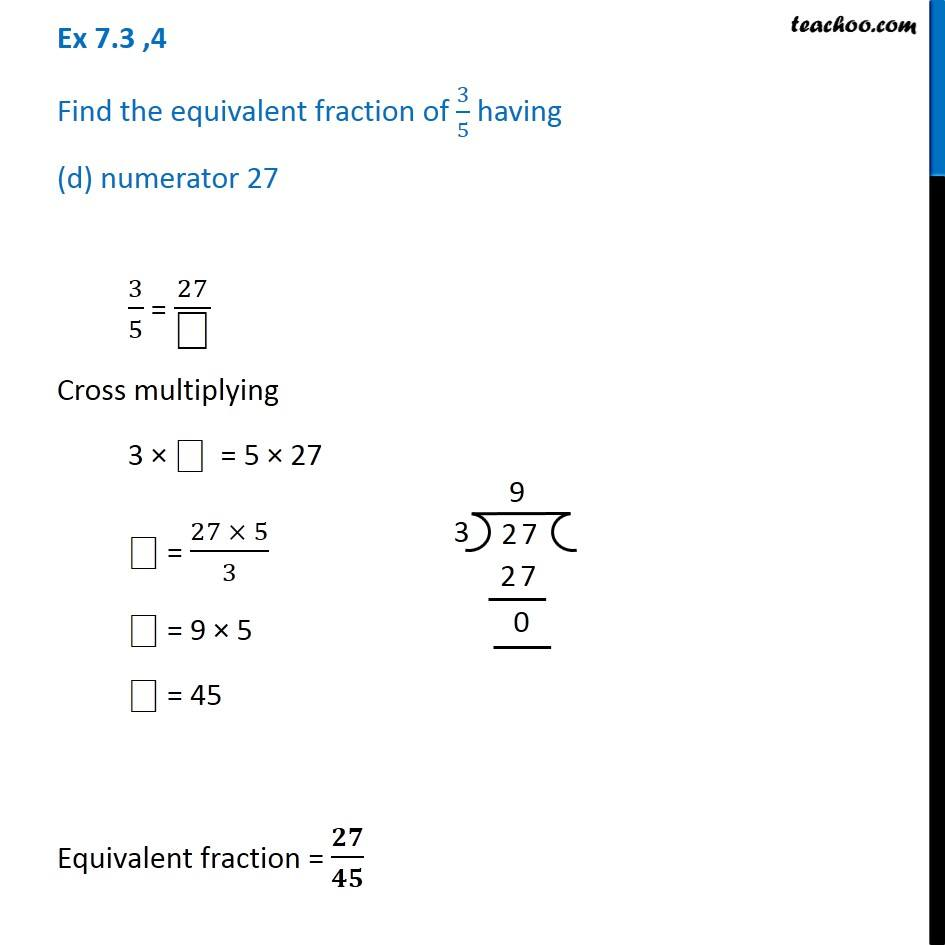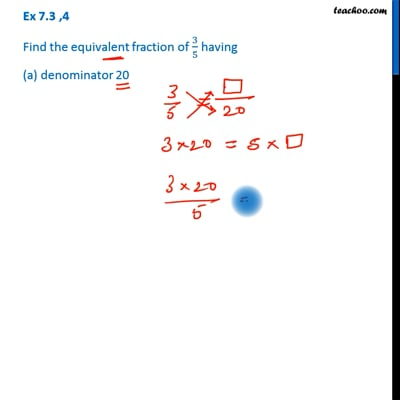This video is only available for Teachoo black users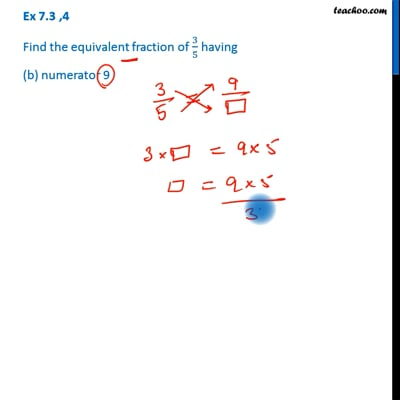This video is only available for Teachoo black users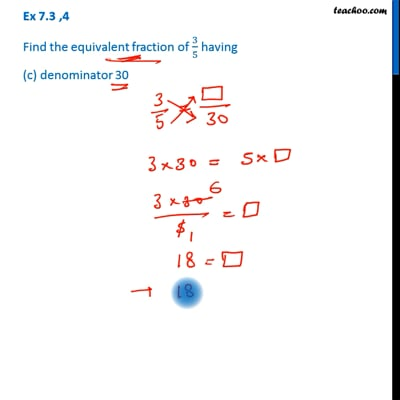This video is only available for Teachoo black users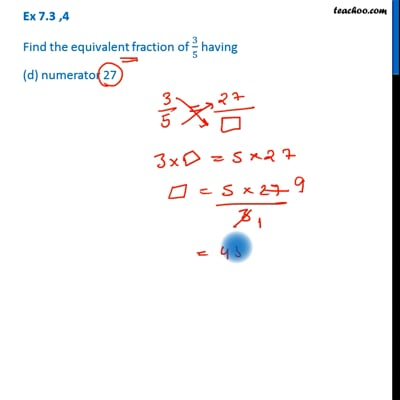This video is only available for Teachoo black users

Learn in your speed, with individual attention - Teachoo Maths 1-on-1 Class

### Transcript

Ex 7.3 ,4 Find the equivalent fraction of 3/5 having (a) denominator 20 3/5 = "⎕" /20 Cross multiplying 3 × 20 = "⎕" × 5 "⎕" = 3/5 × 20 "⎕" = 3 × 4 "⎕" = 12 Equivalent fraction = 𝟏𝟐/𝟐𝟎 Ex 7.3 ,4 Find the equivalent fraction of 3/5 having (b) numerator 9 3/5 = 9/"⎕" Cross multiplying ⎕ × 3 = 9 × 5 "⎕" = (9 × 5)/3 "⎕" = 3 × 5 "⎕" = 15 Equivalent fraction = 𝟗/𝟏𝟓 Ex 7.3 ,4 Find the equivalent fraction of 3/5 having (c) denominator 30 3/5 = "⎕" /30 Cross multiplying 3 × 30 = "⎕" × 5 "⎕" = 3/5 × 30 "⎕" = 3 × 6 "⎕" = 18 Equivalent fraction = 𝟏𝟖/𝟑𝟎 Ex 7.3 ,4 Find the equivalent fraction of 3/5 having (d) numerator 27 3/5 = 27/"⎕" Cross multiplying 3 × ⎕ = 5 × 27 "⎕" = (27 × 5)/3 "⎕" = 9 × 5 "⎕" = 45 Equivalent fraction = 𝟐𝟕/𝟒𝟓